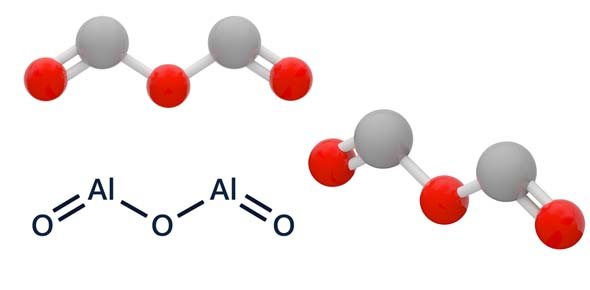What is the sum of the coefficients if the following unbalanced - ProProfs DiscussTopicsMoreProductsMore+ Ask Question

# What is the sum of the coefficients if the following unbalanced equation is balanced?Change Image    Delete

Al + O2 = Al2O3

A. 2
B. 3
C. 4
D. 7
E. 9

This question is part of Chemistry Regents Prep #1
Asked by Kashif, Last updated: Jul 10, 2020

###Request 0FollowShareAnswer AnonymouslyAnswer LaterCopy Link#### E. Stanley

E. Stanley, Technical writer, Indianapolis

The sum of the coefficients is basically the total number of reacting molecules and products formed. However, you need to balance the equation first in order to reach the right value. For that, balance the aluminum with the oxygen and the product side.

This will give use 4 aluminum atoms reacting with 3 oxygen molecules to form 2 molecules of AL2O3. We can easily find the sum by adding all the molecules. 4+3+2=9. The sum of coefficients for this equation is 9. You can find the coefficient for other equations using this method as well. Simply balance the equation and then add the molecules on each side of the equation.#### J.Spencer

Knowledge Enthusiast, Knows A Lot of Stuff.

J.Spencer, Knowledge enthusiast, Tokyo

Given Equation:

Al + O2 ------> Al2O3

Balanced Equation:

4Al + 3O2------> 2Al2O3

The sum of the coefficients is equal to the sum of the number of reactingmolecules and the number of products produced in a balanced chemical equation.

Therefore,

4+3+2= 9.#### John Smith

John Smith9

4(Al) + 3(O2) = 2(Alv2)(Ov3). Coefficients are the numbers in front of the element symbols. Add 4 + 3 + 2 to get 9.Search for Google imagesSelect a recommended image
Upload from your computerCancelSearch for Google imagesSelect a recommended image
Upload from your computerCancelSearch for Google imagesSelect a recommended image
Upload from your computerCancel